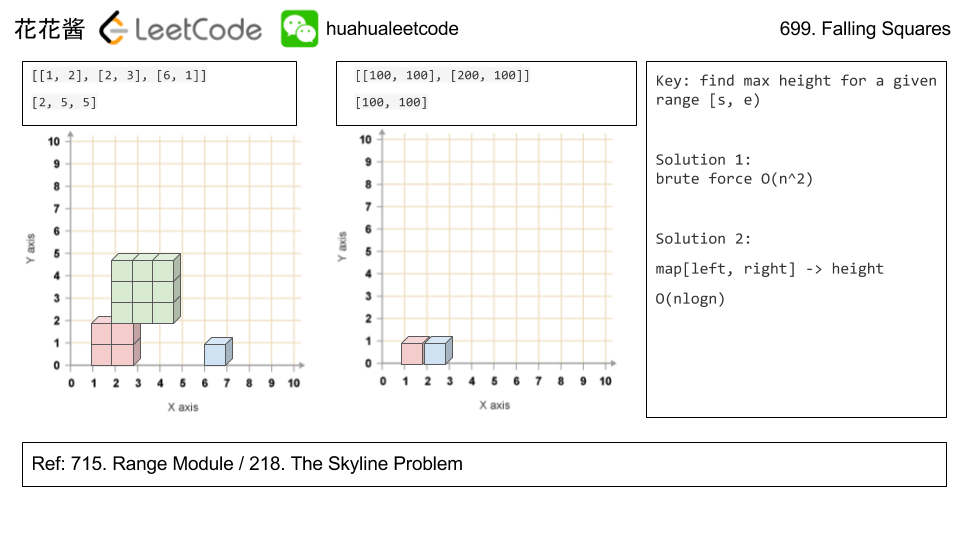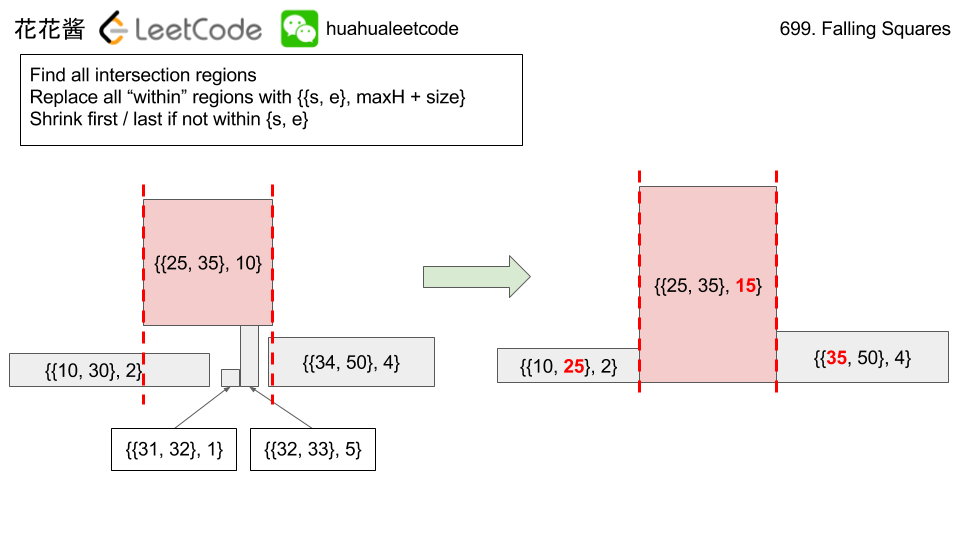Problem:

On an infinite number line (x-axis), we drop given squares in the order they are given.

The i-th square dropped (positions[i] = (left, side_length)) is a square with the left-most point being positions[i] and sidelength positions[i].

The square is dropped with the bottom edge parallel to the number line, and from a higher height than all currently landed squares. We wait for each square to stick before dropping the next.

The squares are infinitely sticky on their bottom edge, and will remain fixed to any positive length surface they touch (either the number line or another square). Squares dropped adjacent to each other will not stick together prematurely.

Return a list ans of heights. Each height ans[i] represents the current highest height of any square we have dropped, after dropping squares represented by positions, positions, ..., positions[i].

Example 1:

Example 2:

Note:

• 1 <= positions.length <= 1000.
• 1 <= positions[i] <= 10^8.
• 1 <= positions[i] <= 10^6.

Idea:

Range query with mapSolution:

C++ map

C++ vector without merge

C++ / vector with merge (slower)

Related Problems:

If you like my articles / videos, donations are welcome.

Buy anything from Amazon to support our website## Be First to Comment

Mission News Theme by Compete Themes.